In this article, I will share my experience with shrinking contour/edge detection models. I will describe in detail model architectures and training experiments that lead to a 6x faster network that underperforms SOTA models by only ~2%.

• Evaluation
• Building Blocks
• Smaller network architectures
• Experiments
• Conclusion

Contour/edge detection state of the art (SOTA) models are cumbersome and slow in most areas of computer vision: semantic segmentation, object detection, human pose estimation. The problem arises when the industry wants to put these models into production. Due to a large number of parameters, they achieve the desired speed only when running on a GPU, which makes them costly. To address this issue there is a lot of research going on to achieve the performance of SOTA models with significantly smaller models. In this article, I will introduce the results of building a small edge detection model that achieves comparable performance with SOTA while being 3 times faster. Specifically, the goal is to achieve the performance of Richer Convolutional Features for Edge Detection RCF (Figure 1) with around 6 times smaller models. Figure 1 can be referred for the precise architecture of RCF and Table 1 can be referred for model size, speed, and accuracy of all models present in the article. Before diving into experiments of training smaller models with knowledge distillation techniques, I will briefly introduce channel pruning and the results of its application to RCF.

## Evaluation

Before introducing the methods and models of channel pruning and knowledge distillation, I will briefly introduce the evaluation procedure which is the same for all experiments. Evaluation metric for edge detection that I will later report in experiments is the optimal dataset scale F1 score (ODS-F). Given an edge probability map, a threshold is needed to produce the binary edge map. Օptimal dataset scale (ODS) employs a fixed threshold for all images in a dataset. F1 score, also named as F-measure is the harmonic mean between precision and recall, namely:

Precision and recall are calculated on the basis of pixels coincidence of ground truth and predicted maps. Before the evaluation non-maximum suppression is applied to output prediction maps. For this purpose, I use this repo, which is a Python implementation of the original MATLAB evaluation codes posted on the BSDS500 home page. Besides it also includes a non-maximum suppression step.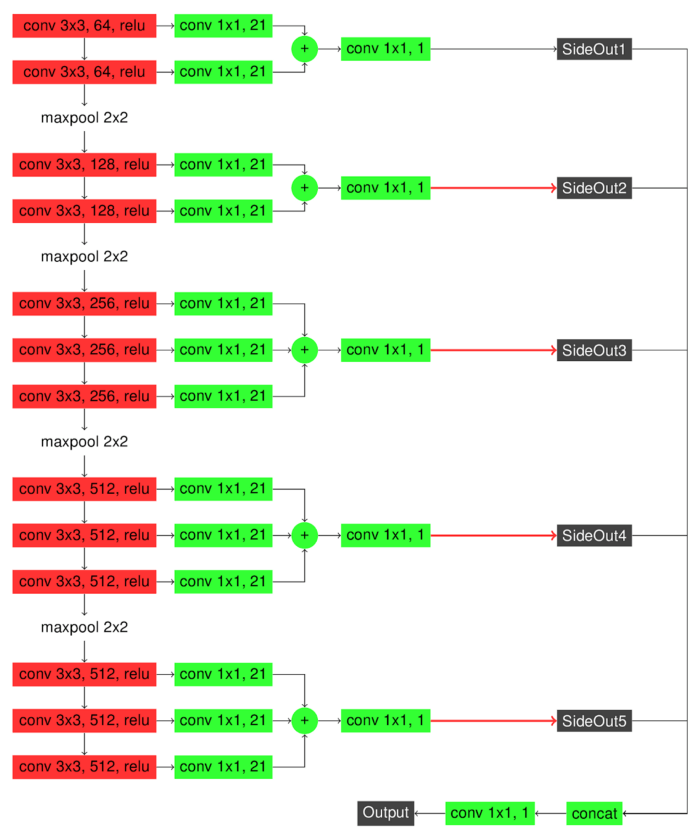Figure 1: RCF network architecture, red arrows correspond to upsampling to input size with bilinear interpolation.

## Building blocks

In deep neural networks repeating structures, which we refer to as building blocks, are very common. There are several common structures used in network architectures that I will present later. You can refer to figures below for the detailed architecture of that building blocks.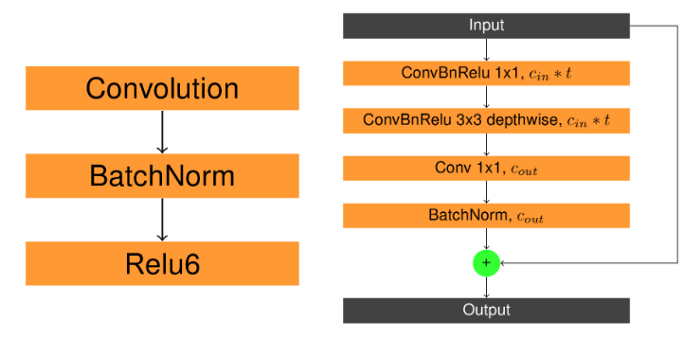Figure 3: Left: ConvBnRelu. Right: InvertedResidualBlock structure, where t is expanding ratio cin is the number of input channels and count is the number of output channels. Depthwise in a ConvBnRelu block means that convolution in that block is depthwise.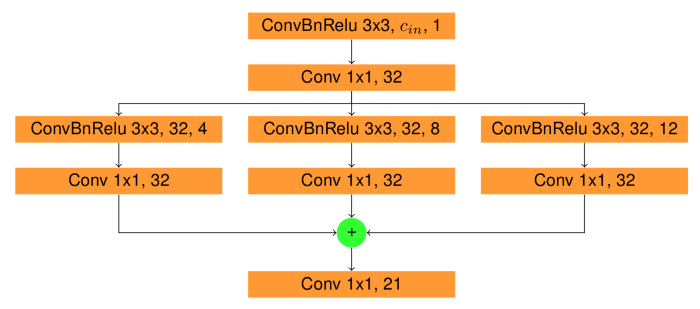Figure 4: Scale Enhancement Module (SEM) structure, convolutional layers in all ConvBnRelu blocks are depthwise, numbers are written after kernel size are the number of output channels and dilation of the convolutional layers respectively. In convolutional layers of all ConvBnRelu blocks, padding is equal to dilation.

## Smaller network architectures

Recent papers in contour/edge detection suggest that fully convolutional encoder-decoder networks show the best performance for the task. So to make a fast performing encoder-decoder network we needed a fast backbone (encoder). MobileNetV2 fulfilled all proposed conditions, both being lightweight due to its depthwise separable convolutions and being a good feature extractor due to its depth. The basic block of decoder is a depthwise convolution followed by pointwise convolution (you can read more about depthwise separable convolutions in this article) followed by upsampling (bilinear interpolation). We employ 5 decoder blocks each of which gets an input the output of the previous block summed with a chosen layer from MobileNetV2. Further, when the training process will be described I will use 2 versions of the model described above: Model (Figure 5) and Side Output Model a.k.a. SO Model (Figure 6). SO Model, besides giving the final output, also gives outputs of all 5 decoder blocks which are referred to as side outputs, while Model only gives one final output. All outputs of each model are used for loss computation with equal weights. Besides introducing model architectures below, a table comparing speed and memory consumption of models is presented.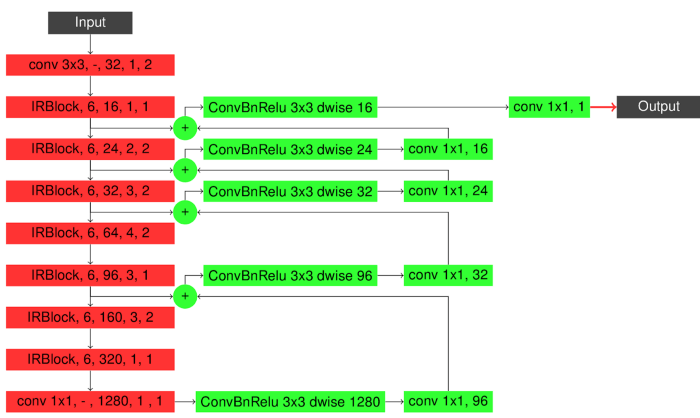Figure 5: Model architecture. IRBlock, t, c, n, s stands for InvertedResidualBlock with expansion factor t, output channel number c, the number of blocks joined sequentially n, the stride of the first convolution in the first block s. Red arrows represent upsampling through bilinear interpolation to the original input size.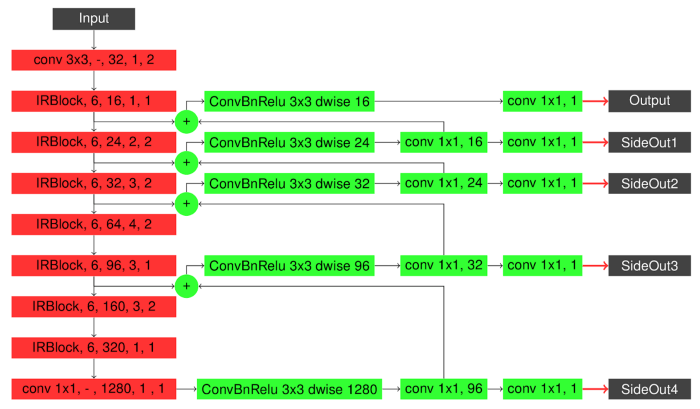Figure 6: SO Model architecture. IRBlock, t, c, n, s stands for InvertedResidualBlock with expansion factor t, output channel number c, number of blocks joined sequentially n, stride of the first convolution in the first block s. Red arrows represent upsampling through bilinear interpolation to the original input size.

## Experiments

For the fairness of experiments hyperparameters of the training are fixed together with data augmentation strategies. Trainings were done for 900 epochs with SGD optimizers. Base learning rates were set to 1e-6 and were decayed every 90 epochs by 0.1. Weight decay and momentum values are set to 5e-4 and 0.9 respectively.

### Experiment 0: Channel Pruning

Channel pruning algorithms work with the following logic: iterating over layers selecting channels that are important according to some criterion, then approximating new weights of the layer usually by linear least squares using accumulated input and output feature maps of the layer from the original unpruned model. These steps can be performed both in training time and inference time, but adding channel pruning steps during training makes it extremely slow, therefore it is usually done during inference time, after which finetuning of the pruned model is done. I have applied the approach presented in arXiv:1707.06168. The channels that will be pruned are selected by Lasso regression, then the new kernels of the layer are approximated with linear least squares using the output of the previous layer that is already pruned and the original output of the layer that is being pruned currently. This approach was originally tested and reported on feedforward networks. Although RCF is not a feedforward network, I applied the method on its backbone (VGG16) and pruned it on Imagenet․ Then I changed the backbone of RCF with the pruned VGG16 and initialized with its fine tuned weights before training it on BSDS500.

While we achieved significant performance improvements of around 2.8x speedup, ODS-F1 score dropped from 0.811532 to 0.726367. So we decided to try the knowledge distillation approach with the goal of preserving the speedup we got with channel pruning while also having smaller performance drop. As you will see later we also managed to further increase the speedup.

### Experiment 1: SO Model

The first experiment is about training SO Model with weighted binary cross-entropy loss function. The total loss function is the sum of all weighted binary cross-entropy losses between 5 outputs of decoder blocks and ground truth edgemap.

*This baseline model achieves 0.735527 ODS-F1 score. Besides the ODS-F1 score I will also present results of each experiment on 3 images from the BSDS500 test set.

### Experiment 2: KD with Hinton’s approach

The second experiment is about training the same SO Model with knowledge distillation described by Hinton et al. Distilling the Knowledge in a Neural Network. The paper introduces the first steps in knowledge distillation, it suggests to use the output of a more sophisticated (teacher) model as a ground truth for a small model (student). So in this experiment the total loss function which is used to train our student model (SO Model) is the sum of weighted binary cross-entropy losses between outputs of SO Model and ground truth as described in the previous experiment and mean squared error loss only between final outputs of student and teacher networks. In all our knowledge distillation experiments Richer Convolutional Features for Edge Detection (Figure 1) network is used as the teacher network. As a result of this experiment, our student model achieved a 0.775432 ODS-F1 score.

### Experiment 3: KD with knowledge adaptor

The third experiment is about application of a more complex knowledge distillation technique to boost the accuracy of the student model. In Knowledge Adaptation for Efficient Semantic Segmentation authors improve a small semantic segmentation model by combination of several knowledge distillation techniques/losses. We employ adaption loss described in the paper. For this purpose the last layer of encoder of both teacher and student networks are projected to the same dimension with the use of global average pooling and fully connected layers. This process is visualized in Figure 10, you can refer to it for more detailed information.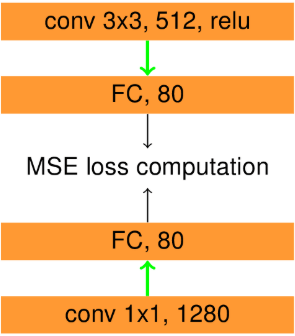Figure 9: conv 3x3, 512, relu is the last convolutional layer of VGG16 and conv 1x1, 1280 is the last convolutional layer of MobilenetV2. Green arrows correspond to global average pooling operation.

After they are in the same dimension a mean squared error loss is calculated between them. We train the Model with cross entropy + 1e4 * adaptation loss. This model achieves a 0.787301 ODS-F1 score.